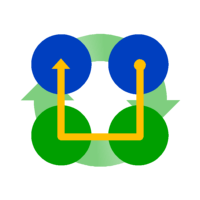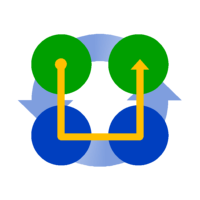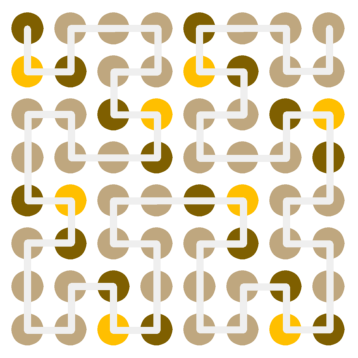# Visual Art Meets Maths / Computer Science

Recently our Auckland office had a new art installation on the wall of one of its meeting rooms. Being a technology company, it was quite apt to make it an array of dots that predictably spelt 'Intergen' in binary (albeit all-caps).

Here's what it looks like below:What I noticed, being uppercase, was that the first three bits were always the same - which is in stark contrast to the pattern formed by the remaining bits. Also noticing that the art was eight bytes (forming a square grid) gave me an idea: Hilbert curves.

Rather than serialising the bits across each row from top to bottom, these bits could be serialised along the path of a Hilbert curve. So how do we construct this Hilbert curve?

A Hilbert curve is a fractal in similar vein to a Koch curve, but constructed in a way that 'fills space' instead of substituting a segment with a copy of the whole structure. What this means is that we define basic components, so for the Hilbert curve we'll have a Clockwise Spin and an Anticlockwise Spin component:We also have logical rules for how each component is recursively composed of sub-components, so we have two different 1st-order Hilbert curves (ignoring effects of rotation):As you can see, a component would occupy one cell, i.e. one dot in the artwork representing one bit. Going to the next level (2nd-order, etc.) means that this cell is divided into four. Following the path according to the direction rotation means that the sub-components are defined in the order 1-0-0-1 (where 1 indicates the sub-component is different to the parent component type). Once a sub-component has been 'rendered', the next component to render is determined by the type of parent component.

For an 8x8 grid, we only need to do this to the 3rd-order, yielding the curve below. This starts with an Anticlockwise spin as the 1st-order curve, starting in the top-left corner. The three most-significant bits for each character form a different pattern, though it isn't so bold as the straight grid before.So all that's left is to follow the path and fill in the remaining bits, to achieve the final result: